# Fractions to whole numbers (3.NF.3C)

##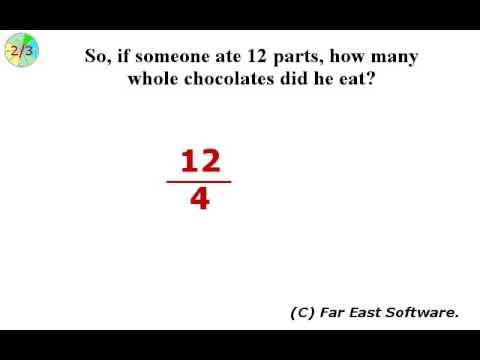By Far East Software

Express whole numbers as fractions, and recognize fractions that are equivalent to whole numbers.# Multiplying Fractions by Whole Numbers 9-9

##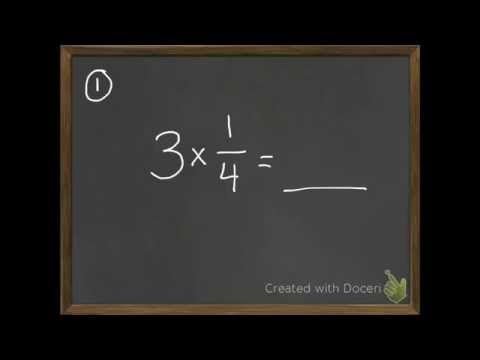By David Horan

multiply a fraction with a whole number# Multiplying Fractions by Whole Numbers 9-9

##By David Horan

multiply a fraction with a whole number.# Multiplying Fractions by Whole Numbers

##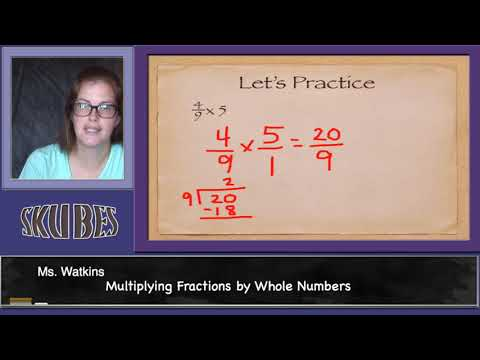By Skubes ed

Understand a multiple of a/b as a multiple of 1/b, and use this understanding to multiply a fraction by a whole number.# [3.NF.3c-1.0] Whole Numbers as Fractions - Common Core Standard

##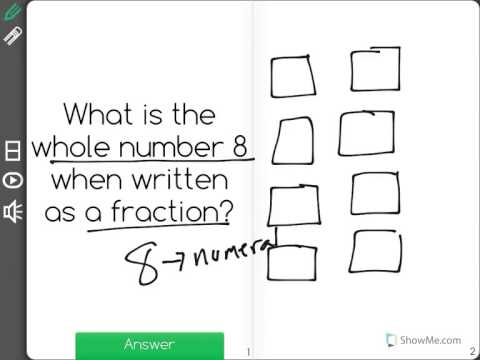By Freckle education

Explain equivalence of fractions in special cases. Express whole numbers as fractions, and recognize fractions that are equivalent to whole numbers.# Dividing whole numbers by unit fractions

##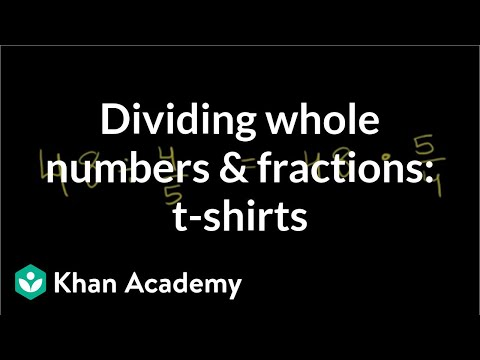By Khan Academy

Dividing a whole number by a fraction? Turn the tables and multiply by the reciprocal of the fraction. Boom!# Multiplying Fractions by Whole Numbers

##By Skubes ed

Understand a multiple of a/b as a multiple of 1/b, and use this understanding to multiply a fraction by a whole number# Multiplying fractions by whole numbers 4.NF.B.4 4.NF.4

##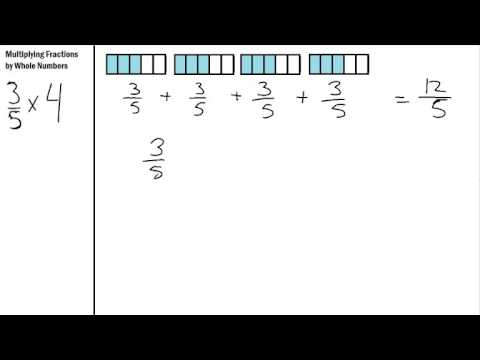By S Hart

Multiplying Fractions by Whole numbers using repeated addition# Visually understanding multiplying fractions and whole numbers

##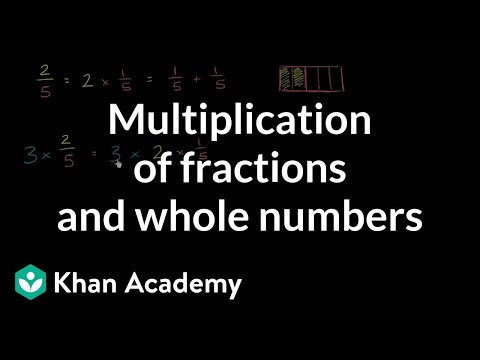By Khan Academy

Here we have a nice visual explanation of how a mixed number is really a multiplication of a fraction and a whole number.# Multiplying fractions by whole numbers word problem | Math | 4th grade | Khan Academy

##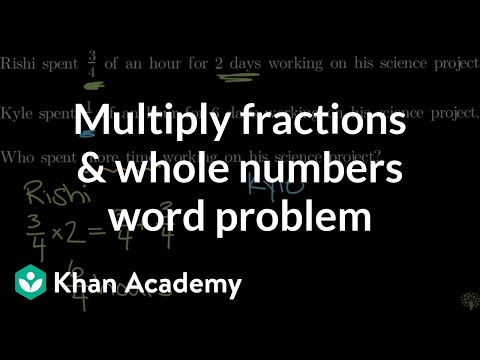By Khan Academy

solves a word problem that involves multiplying a fraction by a whole number# Multiplying fractions by whole numbers word problem | Math | 4th grade | Khan Academy

##By KhanAcademy

solves a word problem that involves multiplying a fraction by a whole number# Learn Fractions - Adding and subtracting fractions with whole and mixed numbers

##By Appuseries

Learn Fractions - Adding and subtracting fractions with whole and mixed numbers ' a feature in the APPU Series.# Subtracting Fractions from a Whole Number

##By Davitily

In this short video students can learn how to subtract fractions from a whole number. The instructor uses computer software to demonstrate. Only one example is used in this video: 3-1/16.# Conceptual understanding of multiplying fractions and whole numbers | Khan Academy

##By Khan Academy

Here we have a nice visual explanation of how a mixed number is really a multiplication of a fraction and a whole number.# Equivalent fractions and different wholes

##By Khan Academy

Learn how two fractions are equivalent only if they refer to the same whole. For example, 1/2 of a small circle is not the same as 1/2 of a big circle.# Dividing whole numbers by unit fractions

##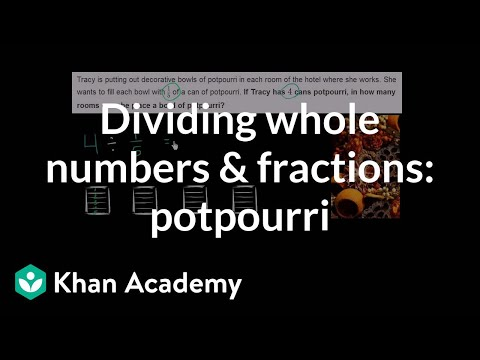By Khan Academy

Solve a word problem by dividing a whole number by a fraction. The example used in this video is 4 ï¿½ï¿½ï¿½ï¿½ï¿½ï¿½ï¿½ï¿½ï¿½ï¿½ï¿½ï¿½ 1/5.# Dividing unit fractions by whole numbers

##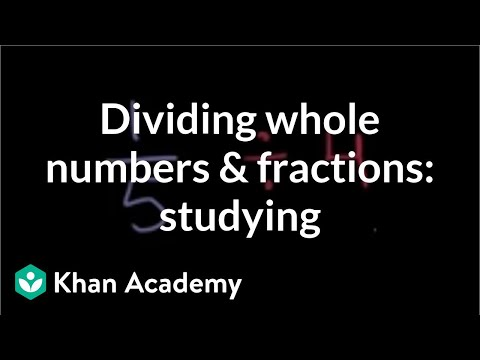By Khan Academy

Solve a word problem by dividing a fraction by a whole number. The example used in this video is 1/5 ï¿½ï¿½ï¿½ï¿½ï¿½ï¿½ï¿½ï¿½ï¿½ï¿½ï¿½ï¿½ 4.# Fractions to whole numbers (3.NF.3C)

##By Far East Software

Express whole numbers as fractions, and recognize fractions that are equivalent to whole numbers.# Whole number and fraction multiplication concept | 4th grade | Khan Academy

##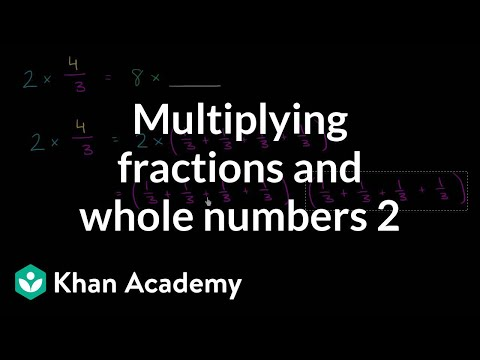By Khan Academy

A mixed number is simply a whole number/fraction multiplication problem. Let us explain.# Dividing whole numbers by unit fractions

##By Khan Academy

Dividing a whole number by a fraction? Turn the tables and multiply by the reciprocal of the fraction. Boom!